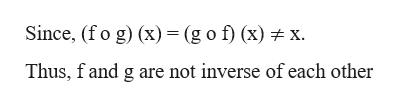# f(x) =x-15 and g(x)=x+14(a) ( f o g )(x)=(b) ( g o f )(x) =(c) thus g(x)=    an inverse function of f(x)

Question
16 views

f(x) =x-15 and g(x)=x+14

(a) ( f o g )(x)=

(b) ( g o f )(x) =

(c) thus g(x)=    an inverse function of f(x)

check_circle

Step 1

Given,

f(x) = x – 15 and g(x) = x + 14

Part a

(f o g) (x) = f(g(x)) = f(x+14) = (x+14) – 15 = x – 1

Part b

(g o f) (x) = g(f(x)) = g(x-15) = (x-15) + 14 = x – 1

Step 2

Part c

...help_outlineImage TranscriptioncloseSince, (fo g) x) (g o f) (x) x Thus, fand g are not inverse of each other fullscreen

### Want to see the full answer?

See Solution

#### Want to see this answer and more?

Solutions are written by subject experts who are available 24/7. Questions are typically answered within 1 hour.*

See Solution
*Response times may vary by subject and question.
Tagged in

### Algebra